# Scanline Fill Algorithm

### Part 1: Scanline Fill

The scanline fill algorithm is an ingenious way of filling in irregular polygons. The algorithm begins with a set of points. Each point is conected to the next, and the line between them is considered to be an edge of the polygon. The points of each edge are adjusted to ensure that the point wih the smaller y value appears first. Next, a data structure is created that contains a list of edges that begin on each scanline of the image. The program progresses from the first scanline upward. For each line, any pixels that contain an intersection between this scanline and an edge of the polygon are filled in. Then, the algorithm progresses along the scanline, turning on when it reaches a polygon pixel and turning off when it reaches another one, all the way across the scanline.

There are two special cases that are solved by this algorithm. First, a problem may arise if the polygon contains edges that are partially or completely out of the image. The algorithm solves this problem by moving pixel values that are outside the image to the boundaries of the image. This method is preferable to eliminating the pixel completely, because its deletion could result in a "backwards" coloring of the scanline i.e. pixels that should be on are off and vice versa.

The second case has to do with the concavity of the polygon. If the polygon has a concave portion, the algorithm will work correctly. The pixel on which the two edges meet will be marked twice, so that it is turned off and then on. If, however, the polygon is convex at the intersection of two edges, the coloring will turn on and then immediately off, resulting in "backwards" coloring for the rest of the scanline. The problem is solved by using the vertical location of the next point in the polygon to determine the concavity of the current portion. Overall, the algorithm is very robust. It turns out that the only difficulty comes with polygons that have large amounts of edges, like circles and ellipses. Filling in such a polygon would be very costly. Luckily, there is a better way to fill circles and ellipses. Below is an image of a polygon filled in with scanfill.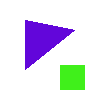### Part 2: Circle and Ellipse Fills

Circles and ellipses are considerably easier to fill than other polygons. These figures are also filled as they are drawn. A pair of boundary pixels sits on each scanline. Simply fill in the pixels between each pair. Since both of these shapes are typically drawn in one quadrant and then reflected around the center point, the space between the pair can be drawn as soon as the two pixels are filled in. Below is an example of an image drawn with the circle adn elipse fills.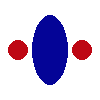### Part 3: Drawing Polygons

A funcion called PolyLine was added ot the graphics environment to simplify the creation of polygons. This function takes in a set of points and draws lines betwen consecutive pairs. This function does not assume that he figureis a closed polygon. If the user wants to create a closed polygon, they must reenter the first point at the end of the list. Below is an image of some lines and polygons created with PolyLine.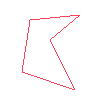### Required Images

#### Box on a Table

The first required image is a scene containing a box on top of a table. This image builds from teh one constructed in the last lab. It incorporates the scanline fill function so that all of the surfaces that were previously open are now filled. The bitmap fill extension is demonstrated in this image, as the fill patterns for the floor and wall.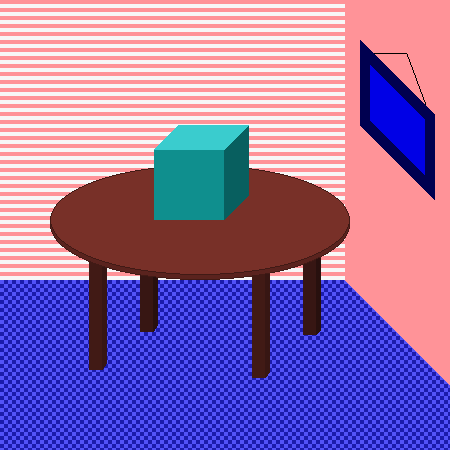#### Strange Polygon

The second required image is an oddly shaped polygon. This polygon exhibits a variety of possible situations in which the fill program may have difficulties.### Questions

1. Who did you work with on this assignment, and what tasks did each of you do?
I worked with Casey Smith, and we each contributed to all of the parts.
2. Is your polygon algorithm consistent with respect to screen coordinate issues, and does it produce rectangles that are the correct area?
Yes. The area of a rectangle created with polyfill is correct. In addition, the polyfill algorithm was adjusted to take into account a shift from a coordinate system centered in the middle of a pixel to a coordinate system centered in the lower lefthand corner of a pixel.
3. How many filled polygons of a reasonable (400 pixels < area < 1000 pixels) size and complexity (5-7 edges) can your algorithm draw in 1 second?
Our program produced 950 polygons per second on Rosemary, a computer with a 440 MHz processor.
4. How many filled circles of a reasonable size (10 pixels < r < 20 pixels) can your algorithm draw in 1 second?
Our circle fill algorithm can draw about 3200 filled circles per second, with an average radius of 14.9 pixels, on a 440 MHz machine (Bay from the Sunlab).
5. If you extended this assignment in any way, describe what you did and how you did it. Include pictures, or links to pictures that show what you did.
We extended the asignment in two ways. First, we implements a bitmap fill as an alternative to the simple polyfill. Below is an image that was created using an 8x8 bitmap.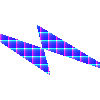In addition, we implemented a gradient fill for polygons. The gradient fill takes in the start and end colors and fills the polygon with a linear progression from the start color to the end color. Below is an image containing a polygon that was filled with the gradient fill.